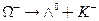Chapter 33, Problem 25PE

Chapter
Section
Textbook Problem

Repeat the previous problem for the decay modeTo determine

(a)

The change in strangeness for the reaction ΩΛ0+K.

Explanation

Given:

Given reaction is, ΩΛ0+K

Calculation:

Strangeness number for Ω=3

Strangeness number for Λ0=1

Strangeness number for K=1

Therefore, for the given reaction,

ΩΛ0+K

S

To determine

(b)

The conservation of charge and baryon number for the reaction ΩΛ0+K while the

lepton numbers are unaffected.

To determine

(c)

To write the equation ΩΛ0+K in terms of quark structure.

Still sussing out bartleby?

Check out a sample textbook solution.

See a sample solution

The Solution to Your Study Problems

Bartleby provides explanations to thousands of textbook problems written by our experts, many with advanced degrees!

Get Started

Find more solutions based on key concepts